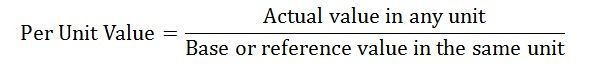# What is Per unit System ?

0
605

## What is Per unit System ?

• Per unit System is the expression of system quantities as fractions of a defined base unit quantity. Calculations are simplified because quantities expressed as per-unit do not change when they are referred from one side of a transformer to the other.
• This can be a pronounced advantage in power system analysis where large numbers of transformers may be encountered.
• Moreover, similar types of apparatus will have the impedances lying within a narrow numerical range when expressed as a per-unit fraction of the equipment rating, even if the unit size varies widely.
• Conversion of per-unit quantities to volts, ohms, or amperes requires a knowledge of the base that the per-unit quantities were referenced to.

### Defination:The per unit value of any quantity is defined as the ratio of actual value in any unit and the base or reference value in the same unit. Any quantity is converted into per unit quantity by dividing the numeral value by the chosen base value of the same dimension. The per unit value are dimensionless.

The base values can be selected arbitrarily. It is usual to assume the base values as given below

• Base voltage = rated voltage of the machine
• Base current = rated current of the machine
• BBase impedance = base voltage /base current
• Base power = base voltage x base current

### Per Unit Calculation :

This is for 3 phase.

VAbase = 1000kVA
Voltage base = 415V
Line voltage = 415V
Power factor = 0.96

I need to find the per unit load current. The way I’ve done it first to get the correct answer is:

Find the load current = I = (VAload)/((root3)*(Line voltage)) = (750kVA)/(root3)*415 = 1043.4

Find base current = (1000kVA)/(root3)*415 = 1391.2

Divide load current by base current = 0.75 p.u.

Calculate angle from power factor = 16.26 degrees.
So load current = (0.75<16.26) p.u. which is correct.

However, using the load VA and the line voltage with the equation S=(root3)VI, with a line voltage as the reference V=1<0 p.u.,

I = (S)/((root3)V)) = (0.75<16.26)/(root3)(1<0) = (0.433<16.26) p.u. which is wrong.

The only thing I can think of is that you’re not meant to divide by (root3) in the above equation, but surely you have to because its 3 phase?